# Energy Test

Created
Best for live in-class or video conferencing lessonsStart teacher-led lesson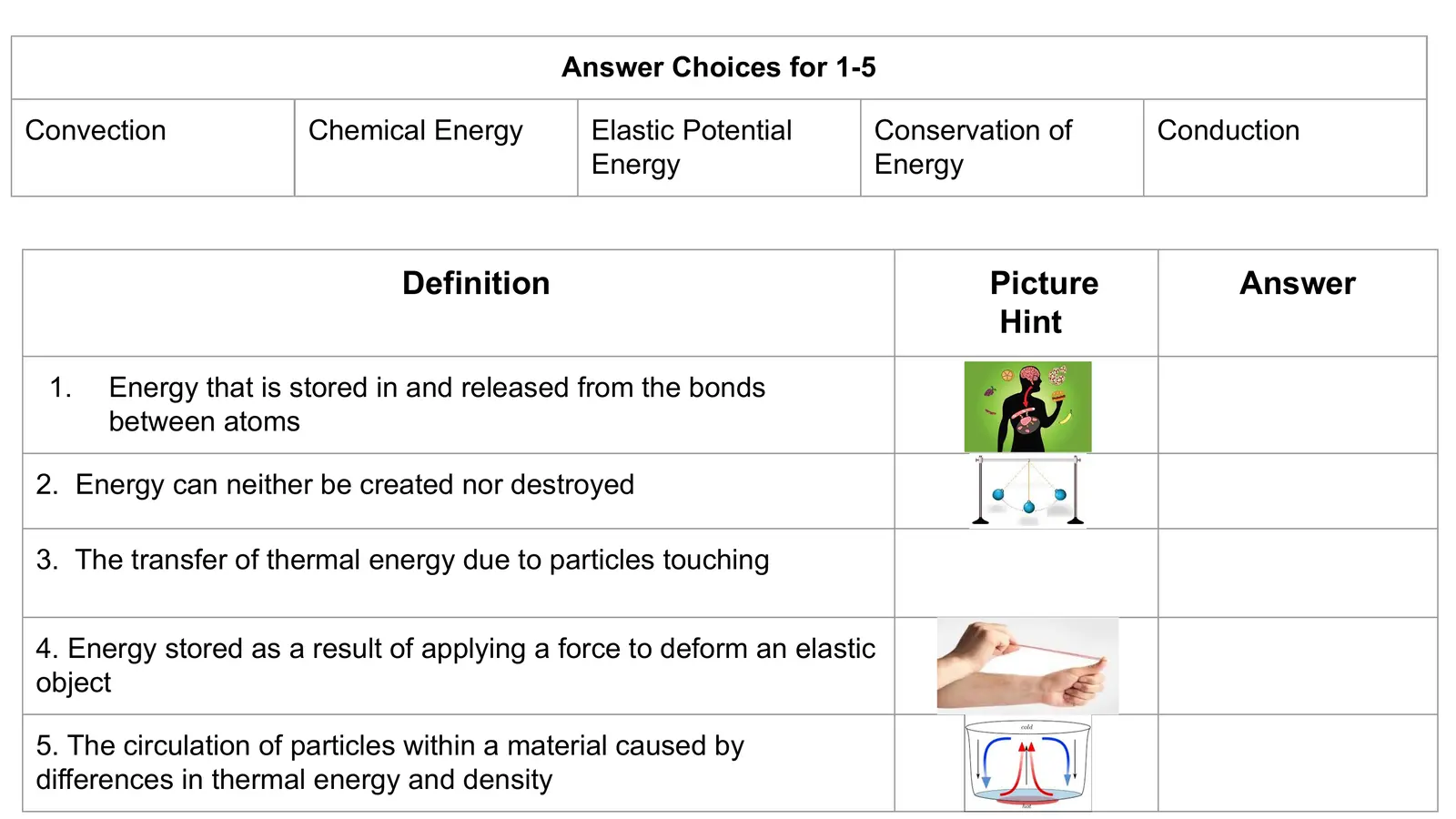Answer Choices for 1-5 Convection Chemical Energy Elastic Potential Conservation of Conduction Energy Energy Definition Picture Answer Hint 1. Energy that is stored in and released from the bonds between atoms 2. Energy can neither be created nor destroyed 3. The transfer of thermal energy due to particles touching 4. Energy stored as a result of applying a force to deform an elastic object 5. The circulation of particles within a material caused by differences in thermal energy and density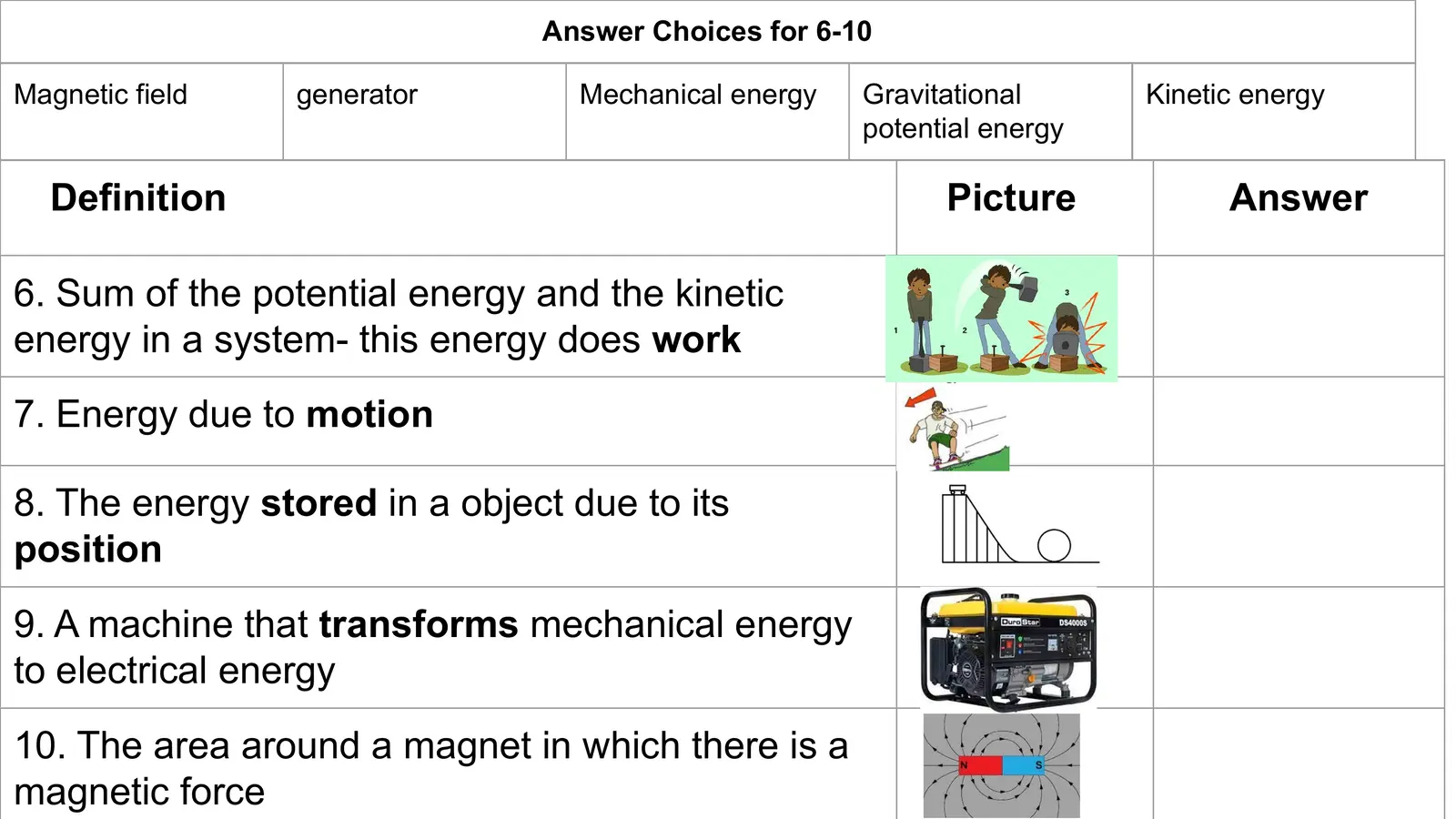Answer Choices for 6-10 Magnetic field generator Mechanical energy Gravitational Kinetic energy potential energy Definition Picture Answer 6. Sum of the potential energy and the kinetic energy in a system- this energy does work 7. Energy due to motion 8. The energy stored in a object due to its position 9. A machine that transforms mechanical energy to electrical energy 10. The area around a magnet in which there is a magnetic force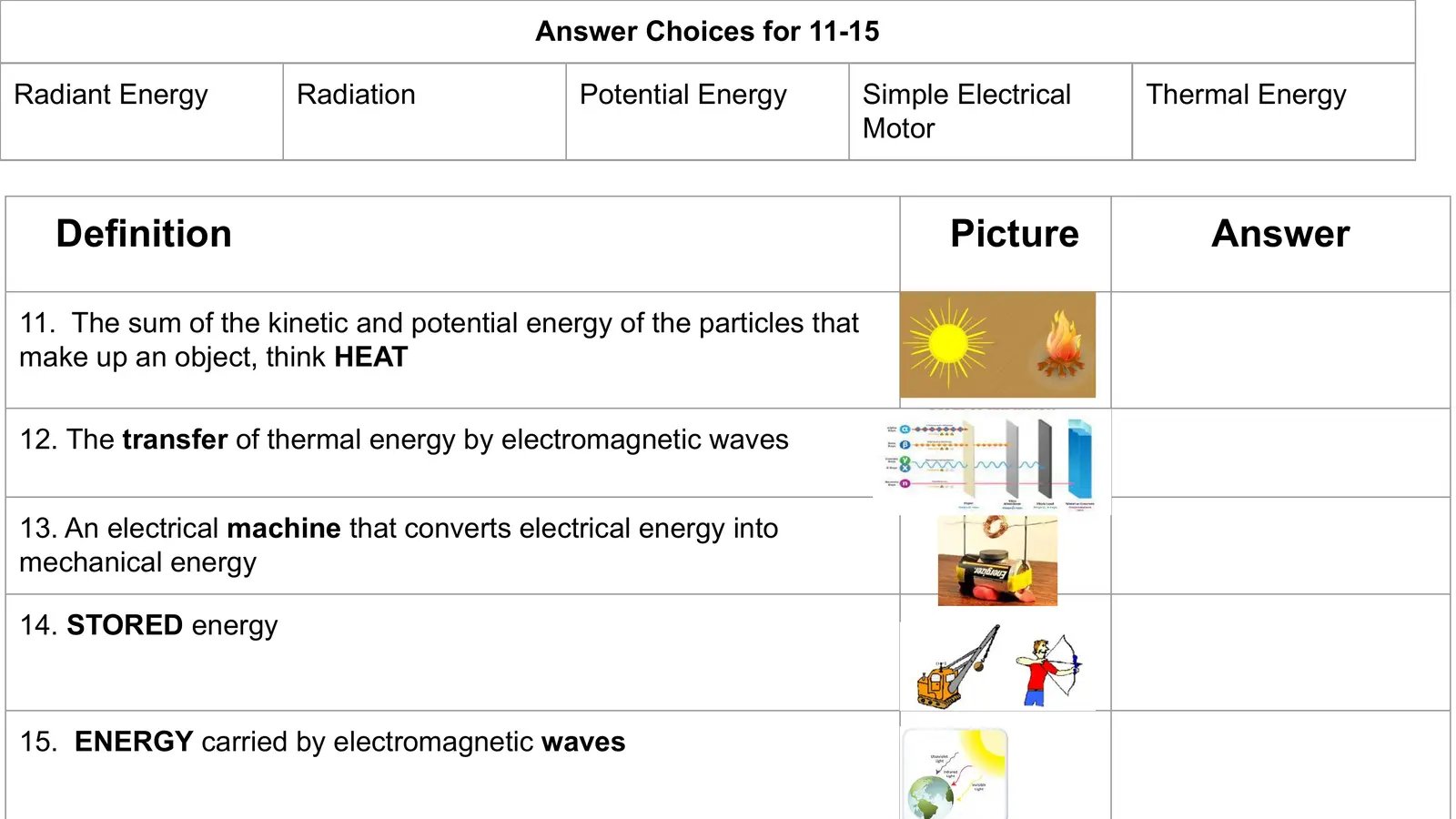Answer Choices for 11-15 Radiant Energy Radiation Potential Energy Simple Electrical Thermal Energy Motor Definition Picture Answer 11. The sum of the kinetic and potential energy of the particles that make up an object, think HEAT 12. The transfer of thermal energy by electromagnetic waves 13. An electrical machine that converts electrical energy into mechanical energy 14. STORED energy 15. ENERGY carried by electromagnetic waves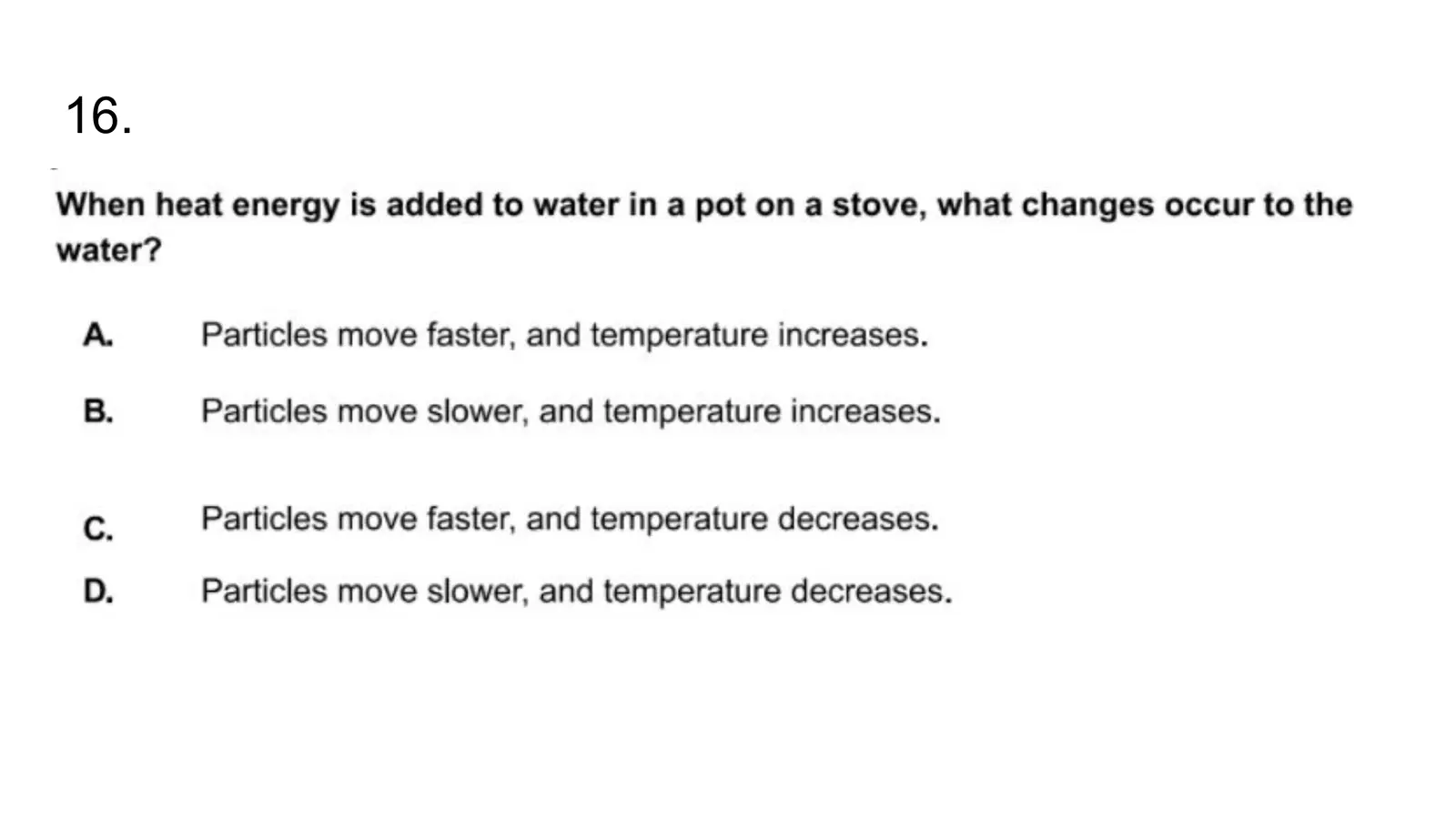16.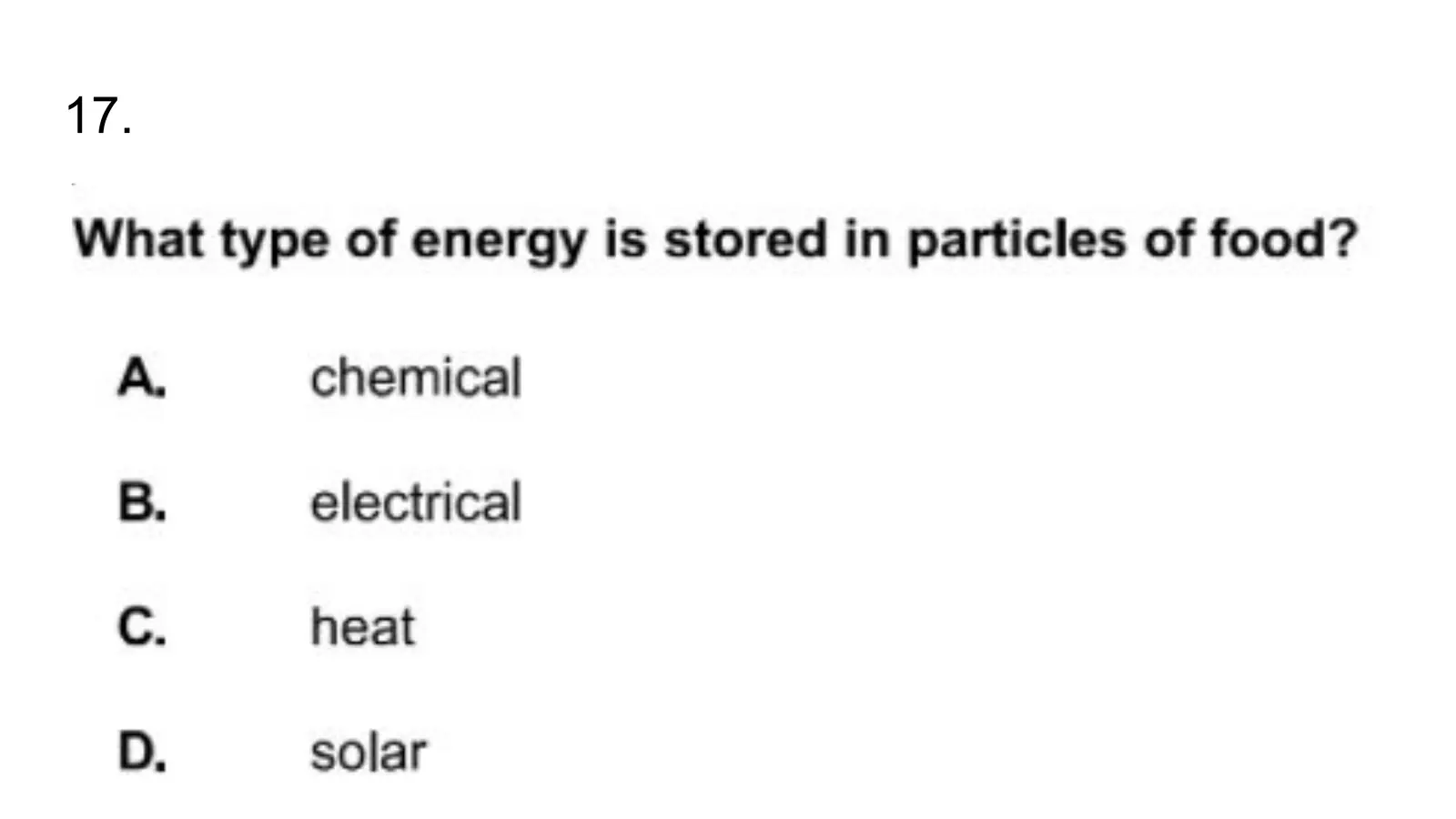17.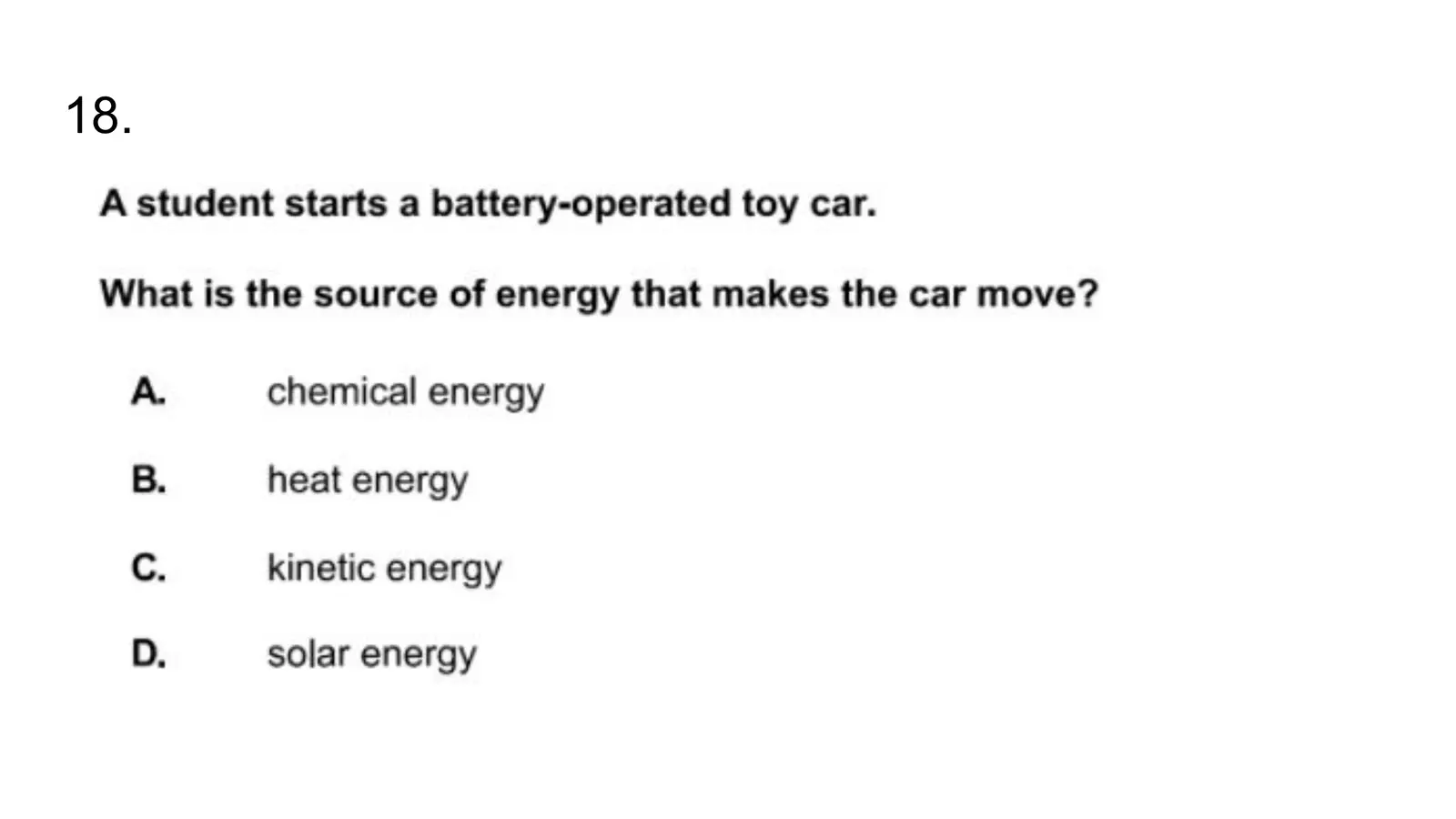18.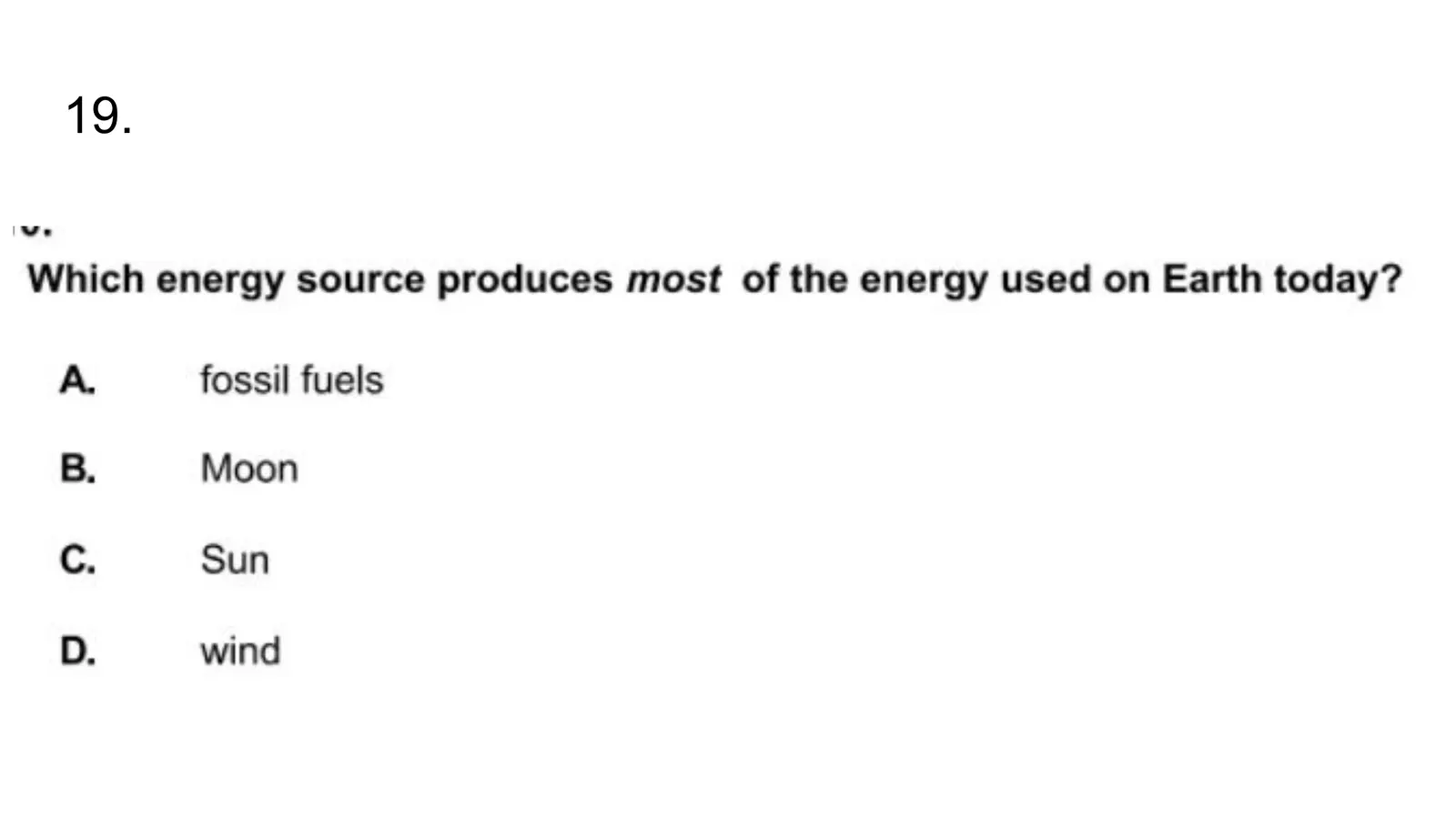19.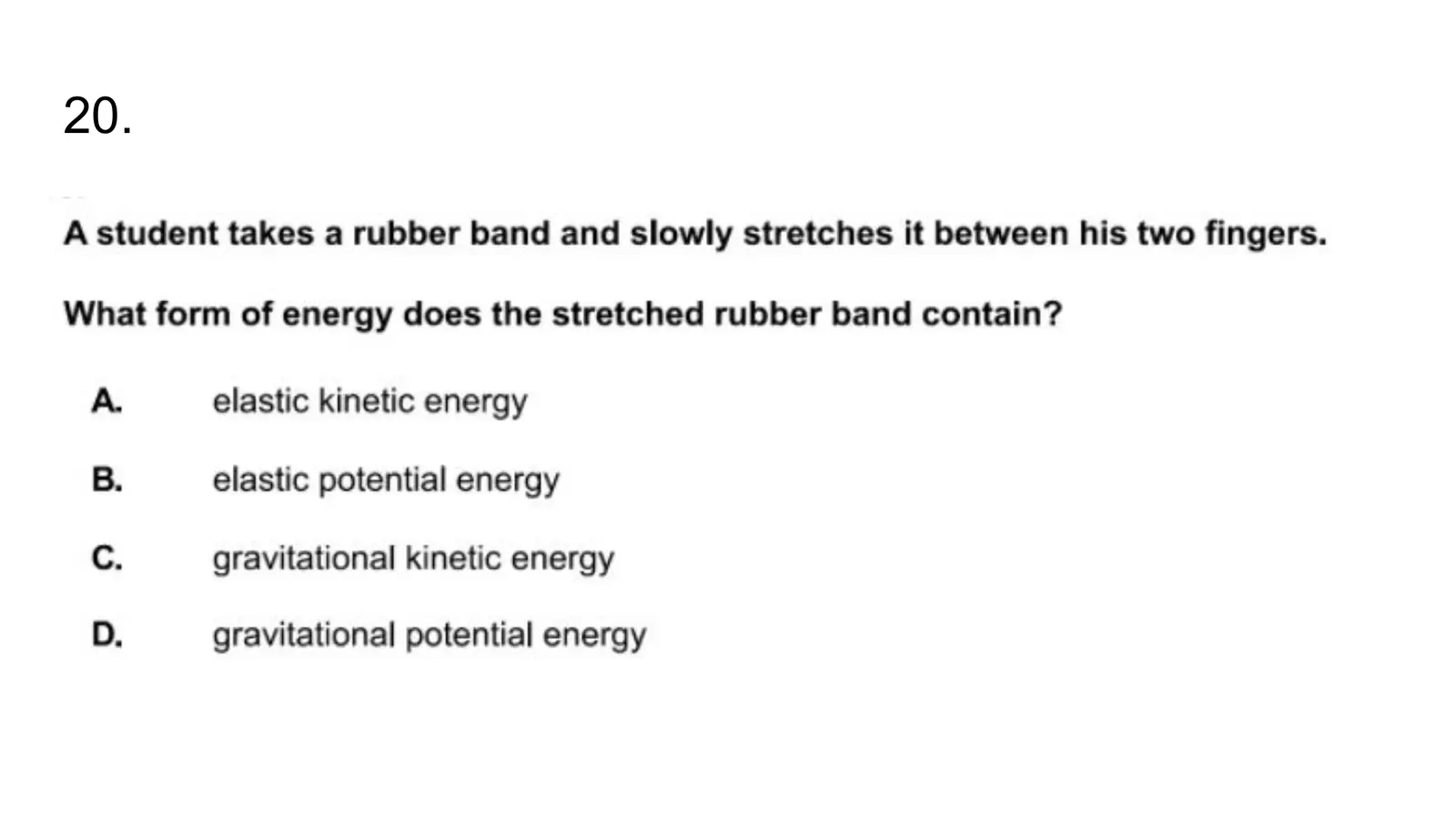20.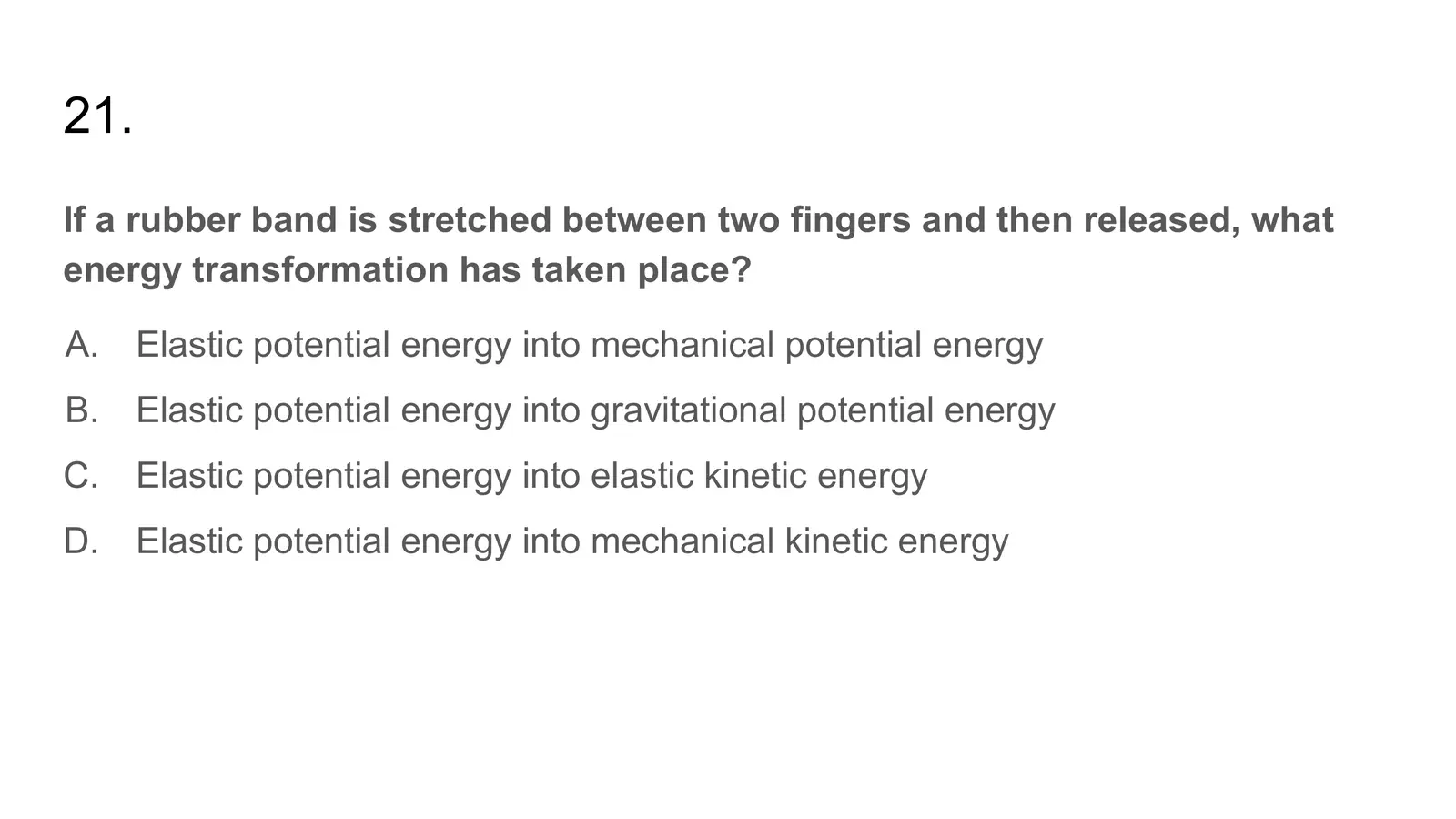21. If a rubber band is stretched between two fingers and then released, what energy transformation has taken place? A. Elastic potential energy into mechanical potential energy B. Elastic potential energy into gravitational potential energy C. Elastic potential energy into elastic kinetic energy D. Elastic potential energy into mechanical kinetic energy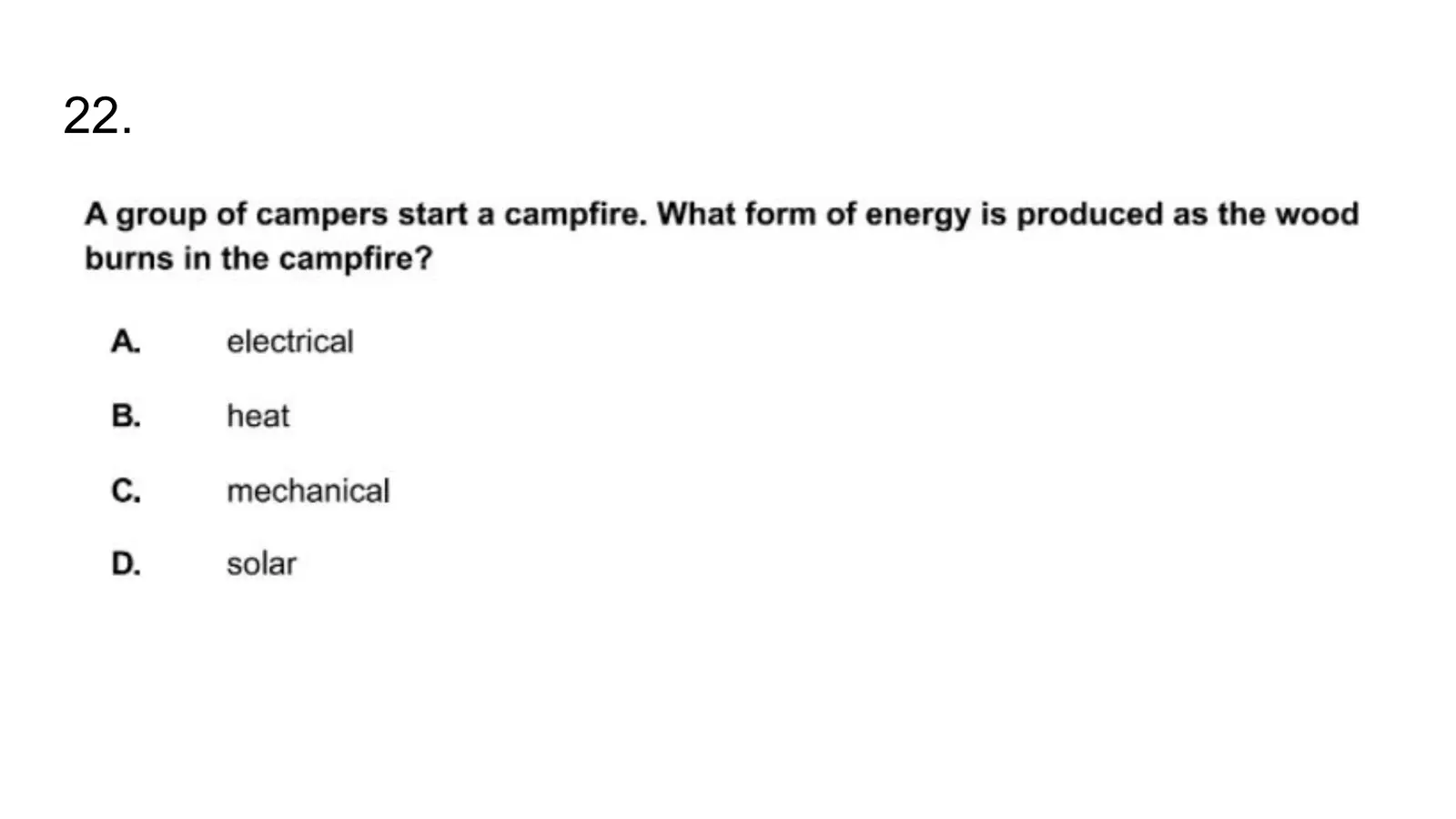22.